10/12/2020

# Reactivity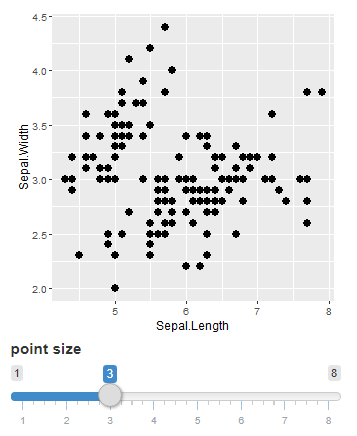1. User changes value of the slider

2. This is flagged by Shiny

3. output$iris_plot is notified that it needs to re-run the code. # Reactive sources and endpointsui <- fluidPage( plotOutput(outputId = "iris_plot", ...), sliderInput(inputId = "slider", ...) ) server <- function(input, output, session) { output$iris_plot <- renderPlot({
ggplot(iris, aes(x = Sepal.Length, y = Sepal.Width)) +
geom_point(size = input$slider) }) }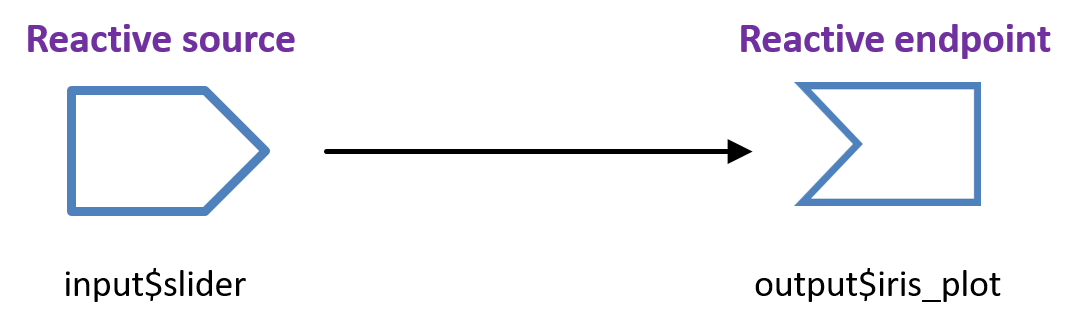# Reactive sources and endpoints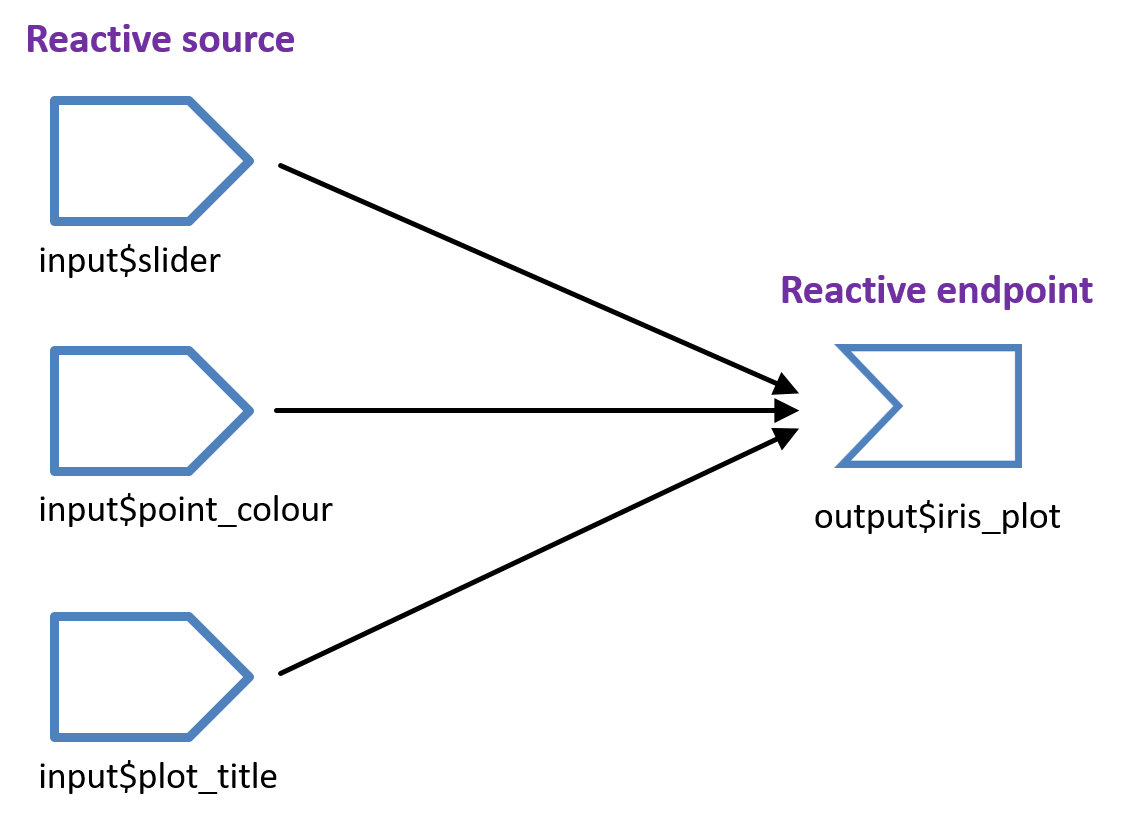# slow app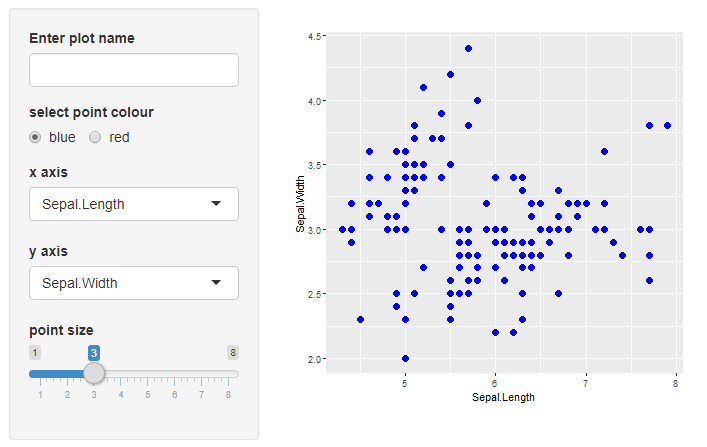# slow app server codeserver <- function(input, output, session) { output$iris_plot <- renderPlot({

point_size <- function(){
Sys.sleep(2)
input$slider + 0.1 } iris %>% ggplot(aes(x = .data[[input$x_attribute]],
y = .data[[input$y_attribute]])) + geom_point(size = point_size(), colour = input$radio_colour) +
ggtitle(input$plot_name) }) # Reactive expressions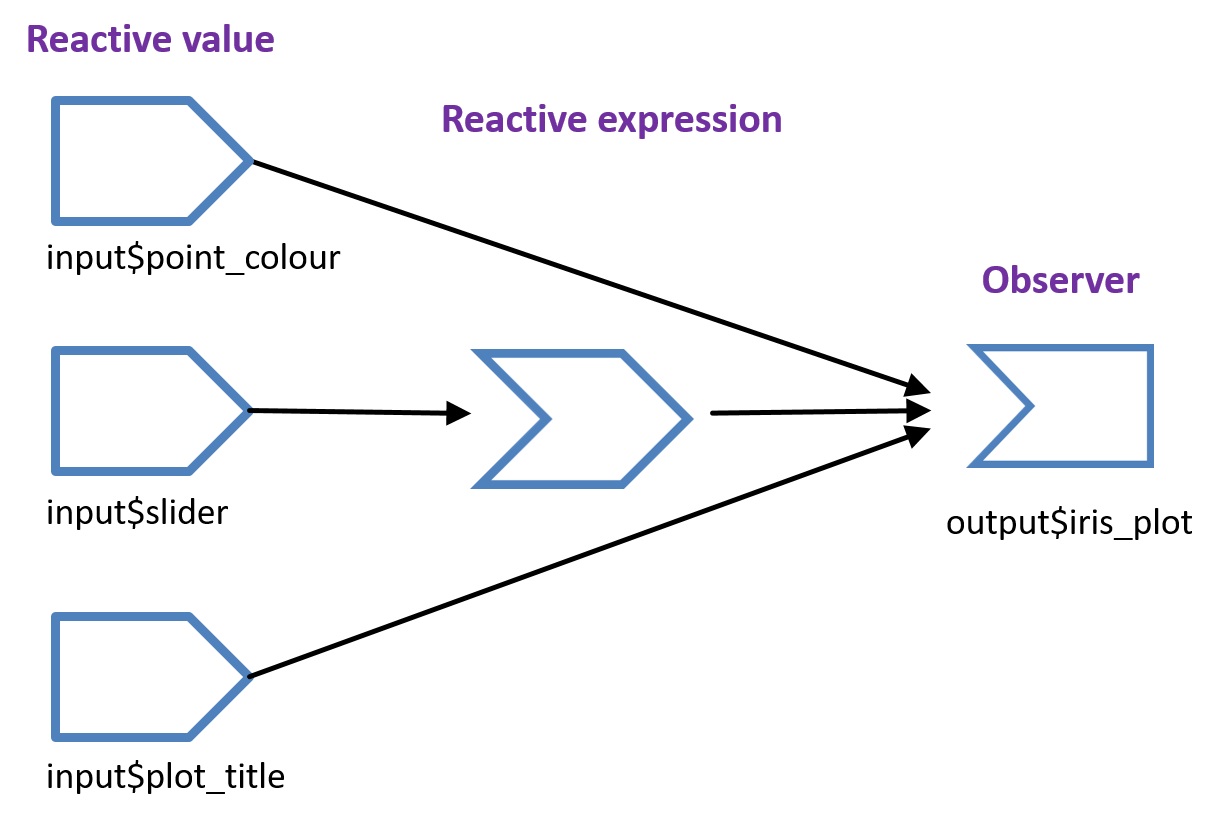Reactive expressions can access reactive values or other reactive expressions and they return a value. They can cache values. # reactive() syntax my_reactive_expr <- reactive({ #code in here…. }) Defining a reactive expression my_reactive_expr() Call it as you would a function point_size <- reactive({ Sys.sleep(2) input$slider + 0.1
})
point_size()

# reactive()

server <- function(input, output, session) {

point_size <- reactive({
Sys.sleep(2)
input$slider + 0.1 }) output$iris_plot <- renderPlot({
iris %>%
ggplot(aes(x = .data[[input$x_attribute]], y = .data[[input$y_attribute]])) +
geom_point(size = point_size(),
colour = input$radio_colour) + ggtitle(input$plot_name)
})
• returns a value
• caches its value
• callable
• lazy – the code doesn’t run until it’s called

Reactive expressions can be called by render functions. Render functions are a special implementation of an observer. They are eager, not lazy and so will re-evaluate the code when a reactive value or expression changes.
There are other observers that aren’t render functions, observeEvent() and observe().

# Faster app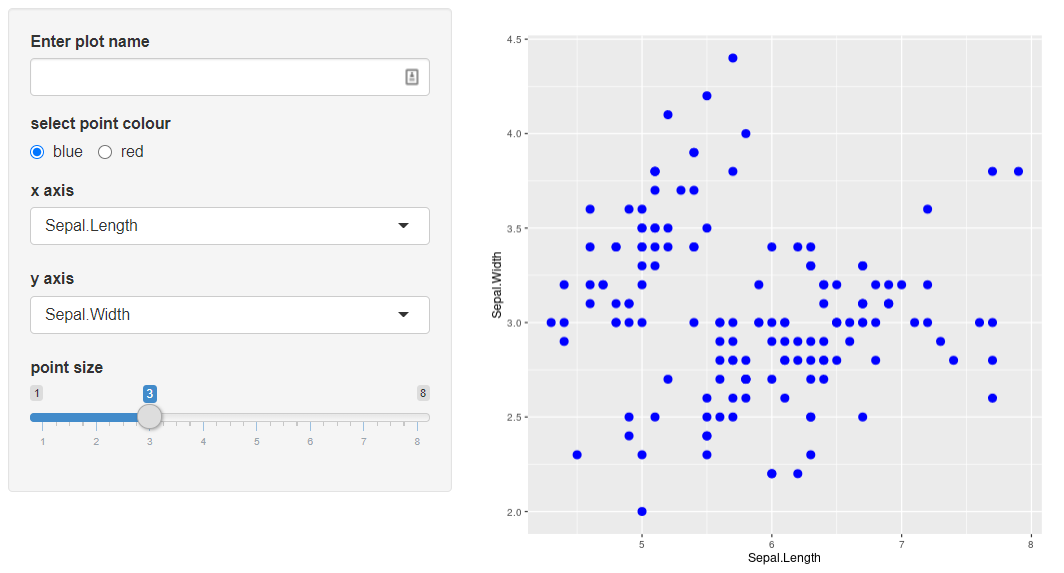# isolate()

• wraps around reactive value or expression
isolate(input$text_field) The value of input$text_field can still be accessed but any changes in text_field will not cause the code to be re-evaluated.

# Example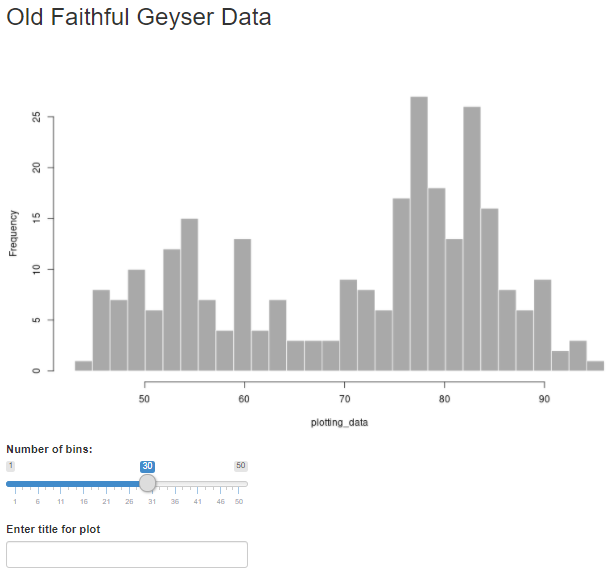# reactive()

Call a reactive expression as you would a function

point_size <- reactive({
Sys.sleep(2)
input$slider + 0.1 }) point_size() # isolate() Wrap an input value or reactive() in isolate() to stop it causing code to be re-evaluated when the value changes. isolate(input$slider)
isolate(point_size())

# Exercises 1 and 2

For each exercise open the app.R file and follow the instructions set out in the comments at the top of the script.

Using a reactive expression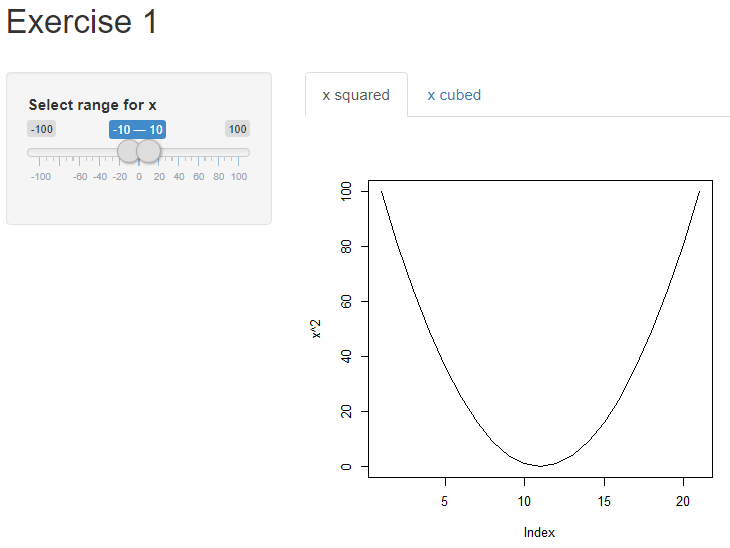Using reactive() and isolate()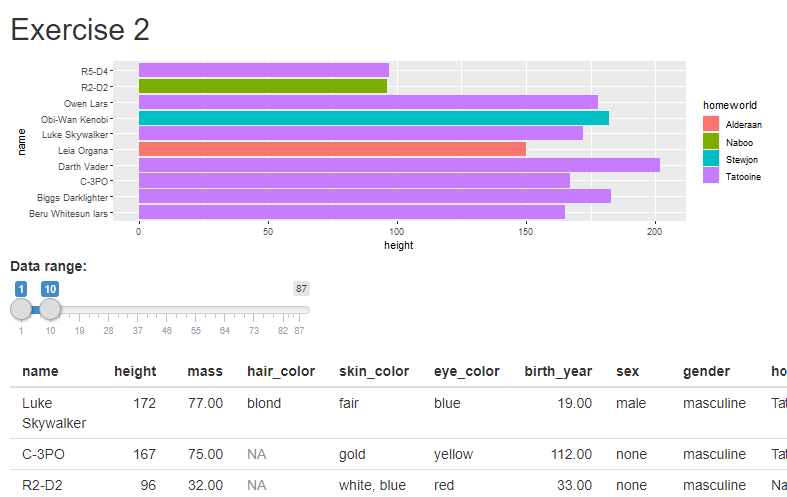# Reactive expressions and observers

Both store expressions that can be executed.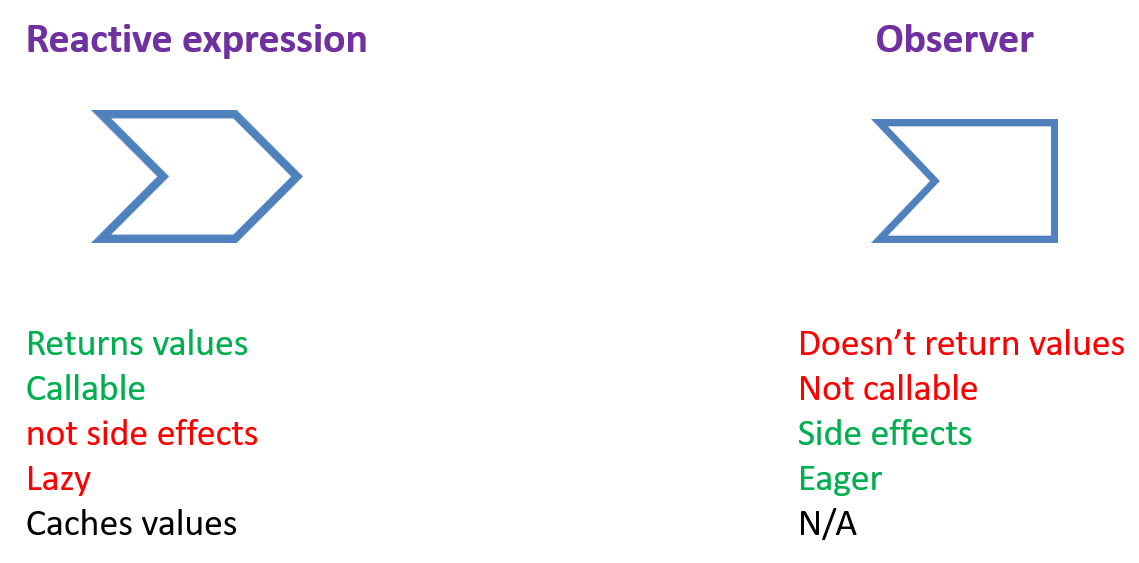Reactive expresssions need an observer as a descendent in order to execute.

reactive() – calculating values without side effects
observe() – performing actions with side effects

# Chick app

Examples of lazy vs eager, reactlog, observeEvent(), eventReactive()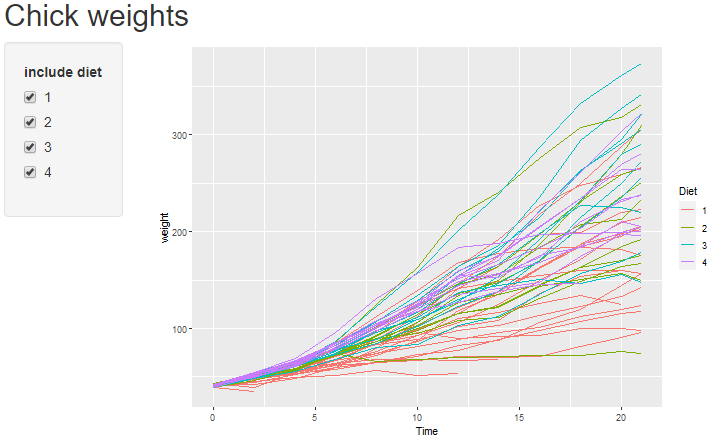# To use the reactlog option insert the following in the app.R script
# after the packages have been loaded
options(shiny.reactlog = TRUE)
# run the app in a browser
# press Ctrl+F3 (Command + F3 for Macs) in the browser to launch the visualisation

# observe functions

observeEvent()

Code will evaluate when reactive values in first argument change, i.e. only when input$update changes. observeEvent(input$update, {
print(paste0("button count = ", input$update)) print(paste0(length(input$selected_diets),
" diets are currently selected"))
})

observe()

The code block will evaluate when any of the reactives change.
This should be used with caution to avoid inefficiencies.

observe({
print(paste0("button count = ", input$update)) print(paste0(length(input$selected_diets),
" diets are currently selected"))
})

# Reactive expressions

reactive()

Reactive expression

data_subset <- reactive({

ChickWeight %>%
filter(Diet %in% input$selected_diets) }) eventReactive() Reactive expression that is only evaluated when reactive value in first argument is invalidated data_subset <- eventReactive(input$update, {

ChickWeight %>%
filter(Diet %in% input$selected_diets) }) # Reactive values reactiveVal() Single reactive value that can be set and updated rv <- reactiveVal(25) rv(30) # to modify value from an observer rv() # to access value reactiveValues() A list of reactive values that can be set and updated (the input object is a reactiveValues object) my_rv <- reactiveValues(x = 5, y = 10) my_rv$x <- 7   # to modify value from an observer
my_rv$y <- 20 my_rv$x   # to access value

# Reactive values app

app on server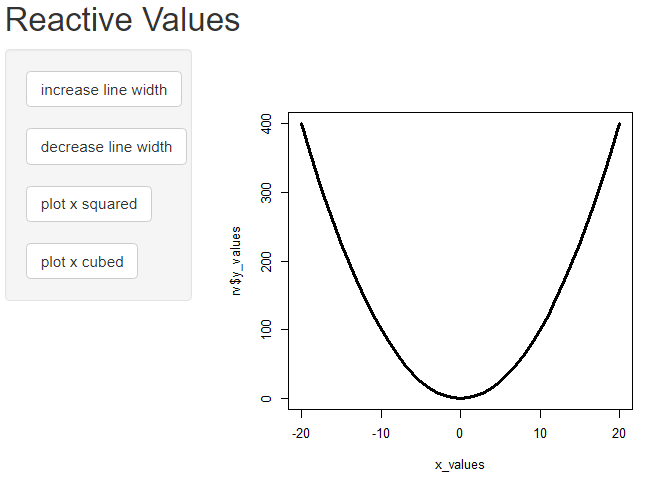# code for reactive values app

### reactiveVal()

x_values <- -20:20
server <- function(input, output) {
line_width <- reactiveVal(3)
y_values <- reactiveVal(x_values)

observeEvent(input$lwd_increase, { line_width(line_width()*1.5) }) observeEvent(input$lwd_decrease, {
line_width(line_width()/1.5)
})
observeEvent(input$plot_x_squared, { y_values(x_values^2) }) observeEvent(input$plot_x_cubed, {
y_values(x_values^3)
})
output$x_plot <- renderPlot({ plot(x_values, y_values(), type = "l", lwd = line_width()) }) } ### reactiveValues() x_values <- -20:20 server <- function(input, output) { rv <- reactiveValues( line_width = 3, y_values = x_values^2 ) observeEvent(input$lwd_increase, {
rv$line_width <- rv$line_width*1.5
})
observeEvent(input$lwd_decrease, { rv$line_width <- rv$line_width/1.5 }) observeEvent(input$plot_x_squared, {
rv$y_values <- x_values^2 }) observeEvent(input$plot_x_cubed, {
rv$y_values <- x_values^3 }) output$x_plot <- renderPlot({
plot(x_values,
rv$y_values, type = "l", lwd = rv$line_width)
})
}

# Syntax recap

reactive()

Reactive expression

data_subset <- reactive({
# code here that produces a return value
ChickWeight %>%
filter(Diet %in% input$selected_diets) }) data_subset() # call it as you would a function eventReactive() Reactive expression that is only evaluated when reactive value in first argument is invalidated. data_subset <- eventReactive(input$update, {
# code here that produces a return value
ChickWeight %>%
filter(Diet %in% input$selected_diets) }) data_subset() # call it as you would a function reactiveVal() Single reactive value that can be set and updated rv <- reactiveVal(25) # create rv(30) # set (usually from observer) rv() # get reactiveValues() A list of reactive values that can be set and updated my_rv <- reactiveValues(x = 5, y = 10) # create my_rv$x <- 7   # set (usually from observer)
my_rv$y <- 20 # set (usually from observer) my_rv$x        # get

# Syntax recap

observeEvent()

observeEvent(input$slider, { # this code will execute when input$slider changes,
# does not return a value
})

observe()

observe({
# this code will execute when any reactive values or expressions
# within it change, does not return a value
})

isolate()

# wrap around a reactive value or expression to return a non-reactive copy
isolate(input\$var)

# Reactive expressions and observers

Both store expressions that can be executed.Reactive expresssions need an observer as a descendent in order to execute.

reactive() – calculating values without side effects
observe() – performing actions with side effects

# Updating UI components

UI inputs may need to be updated after user actions. There are a set of update functions available.

update[inputType]
    updateSelectInput(session, id, choices = c("x", "y", "z"))
updateSliderInput(session, id, min = 10, max = 50, step = 5)

# Deploying a shiny app

• Develop locally, deploy when ready
• A Shiny server - make sure required packages are installed on the server
• shinyapps.io https://www.shinyapps.io/

# Exercises 3 and 4

### Using eventReactive()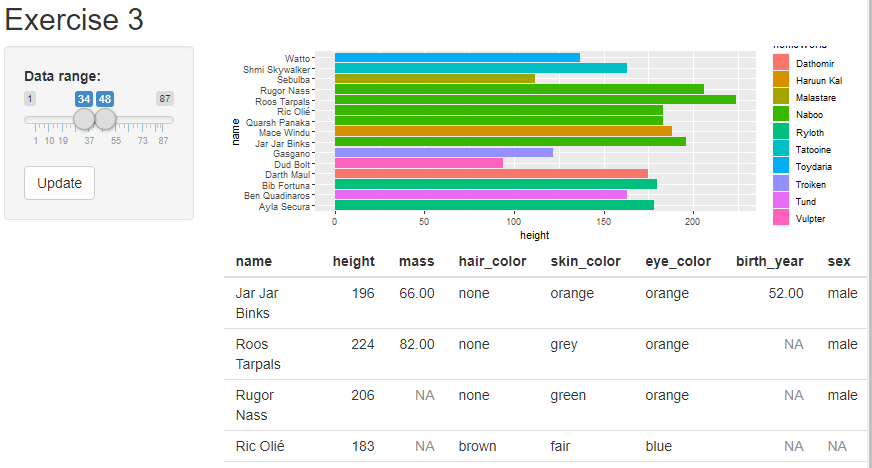### Exercise 4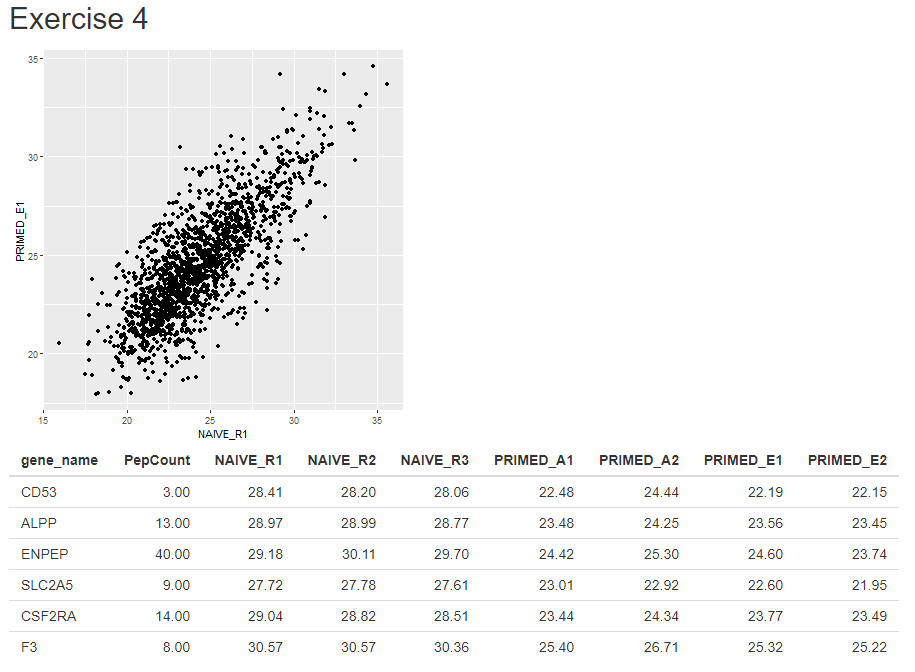# Resources

https://shiny.rstudio.com/articles/understanding-reactivity.html

https://mastering-shiny.org/ early online version of book by Hadley Wickham

https://resources.rstudio.com/shiny-developer-conference/shinydevcon-reactivity-joecheng-part-1-1080p Talk by Joe Cheng about reactivity